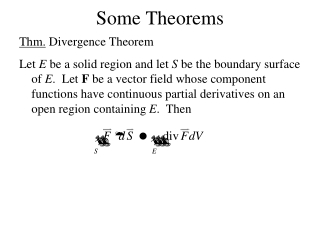Download PresentationSome Theorems

# Some Theorems

Download Presentation## Some Theorems

- - - - - - - - - - - - - - - - - - - - - - - - - - - E N D - - - - - - - - - - - - - - - - - - - - - - - - - - -
##### Presentation Transcript

1. Some Theorems Thm. Divergence Theorem Let E be a solid region and let S be the boundary surface of E. Let F be a vector field whose component functions have continuous partial derivatives on an open region containing E. Then

2. Ex. Evaluate where F = xi + 2yj + zk and S is the surface of the solid bounded by 2x + 2y + z = 6 and the coordinate planes.

3. Ex. Find the flux of the vector field F = zi + yj + xk over the unit sphere.

4. Ex. Evaluate where F = xyi + (y2 + exz)j + ln(xy)k and S is the surface of the region bounded by z = 1 – x2, z = 0, y = 0, and y + z = 2

5. Thm. Stokes’ Theorem Let S be an oriented piecewise-smooth surface that is bounded by a simple, closed, piecewise-smooth curve C. Let F be a vector field whose components have continuous partial derivatives on an open region in 3 that contains S. Then

6. Ex. Evaluate where F = -y2i + xj + z2k and C is the curve of intersection of the plane y + z = 2 and the cylinder x2 + y2 = 1.

7. Ex. Evaluate where F = -y2i + zj + xk and C is the boundary of the portion of 2x + 2y + z = 6 that lies in the first octant.

8. Ex. Evaluate where F = xzi + yzj + xyk and S is the part of the sphere x2 + y2 + z2 = 4 that lies inside the cylinder x2 + y2 = 1 and above the xy-plane.

9. Line Integrals • If F(x,y) is conservative: • We can change the path • if the path is closed • If C is closed: • (Green’s Theorem)

10. Surface Integrals • If S is closed: • (Divergence Theorem) • If C is the edge of S: • (Stokes’ Theorem)